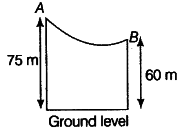# Give an example where potential energy

(i) Define potential energy.
(ii) Give an example where potential energy is acquired by a body due to change in its shape.
(iii) A skier of mass 50 kg stands at A, at the top of a ski jump. He takes off from A for his jump to B. Calculate the change in his gravitational potential energy between A and B.(i) Potential Energy It is the energy possessed by a body by virtue of its position or shape.
(ii) In a toy car, the wound spring possesses potential energy. When spring is released, its potential energy changes into kinetic energy due to which the toy car moves.
(iii) Given, m = 50 kg, h = 75 m, h = 60 m
At point A, PE = mgh = 50 x 10 x 75 = 37500 J
At point B, PE mgh = 50 x 10 X 60 = 30000 J
Change in PE = PE, -PE, = 37500- 30000 = 7500 J